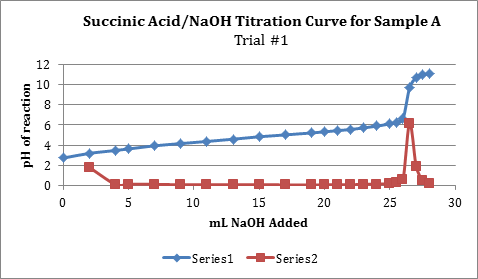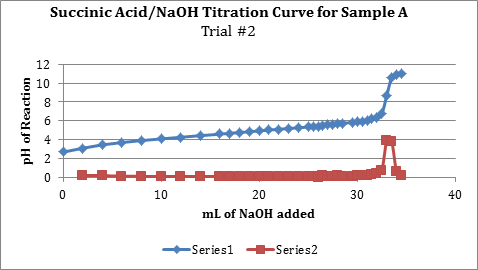# Acid Impurity and Identification Through Molar Mass Calculation

Acid Impurity and Identification Through Molar Mass Calculation
By Sena Contreras

Introduction:
The purpose of part I in the investigation was to determine if two methods of purifying succinic acid produce statistically similar results.  We tested this using a pooled t-test; the null hypothesis being that there is no difference between the samples A and B.  Sample A was purified by the first method, and sample B by the new method.  The purity of each was found through an acid base titration. The objective in the second part of the lab was to calculate the molar mass of an unknown diprotic acid.  The molar mass was found with an acid-base titration and basic stoichiometry.

Procedure:
All glassware used was class A for all parts of the investigation. Basic safety precautions should be taken such as wearing goggles and gloves at all times.

Part I
Two samples of succinic acid, weighing around 0.2 grams each, were dissolved in 50 ml deionized water in separate Erlenmeyer flasks. A buret was rinsed and then filled with standardized NaOH solution around 0.1 M. A pH probe was calibrated with pH 4 and 7 buffers. The pH of the reaction was recorded as NaOH was added. This procedure was repeated for two trials.

Part II
The buret used in part I was refilled with NaOH solution. A 0.2g sample of the unknown acid was weighed and transferred to an Erlenmeyer flask. The sample was dissolved in 10ml-deionized water. Methyl red was used as the indicator. The aqueous unknown was titrated to the equivalence point.

Data/Calculations:
Part I:Note: Series 1 in the graph is the titration curve, while series 2 shows the derivate values or slopes between points in series 1.Note: Series 1 in the graph is the titration curve, while series 2 shows the derivate values or slopes between points in series 1.

 Mass Succinic Acid Volume NaOH usedAt equivalence Volume NaOH used at indicator end point Molarity of NaOH solution Percent purity % 0.2264g 26.5 mL 20 mL 0.1406M 99.10% 0.2036g 33.0 mL 27 mL 0.10163 M 97.26 %

Moles Succinic Acid =
0.0265 L NaOH x (0.1406 mol NaOH/ 1 L) = 0.0037 mol NaOH/2 = 0.0019mol Succinic acid
% Purity= [(0.0019 mol SA x 118.09 g/mol)/0.2264g] x 100 = 99.10 %

 Sample A Succinic Acid Sample B Succinic Acid Mean % purity 93.72 % 94.51% Standard Deviation % purity 16.26 % 10.67% Number of trials 16 9

Spool=Ö[[(16-1)(16.26)2+(9-1)(10.67)2]/(16+9-2)] = 14.56
Sxpool=14.56/[Ö(16×9)/(16+9)] =6.07
tcalc= ½93.72-94.51½/ 6.07 = 0.13
d.o.f =16 + 9 -2 = 23
ttable @ 99%= 2.58
tcalcÐttable  therefore we fail to reject the null. The samples are statistically the same at the 99% confidence interval.

Part II:

 Trial Mass of Unknown Sample Used Volume NaOH used (mL) Calculated Molar Mass of Unknown Acid (g/mol) 1 0.2134 29.4 142.84 g 2 0.2730 37 145.201 g

Molar Mass = [1/ (0.0294 L NaOH) x (0.10163 mol/L) x (1/0.2134g)] x 2 = 142.84 g
Mean molar mass = 144.021g
Standard deviation of molar mass= +/- 1.67g

Discussion:
The pooled t-test compares the two samples that have been purified in different ways and determines weather or not the results are statistically the same at the 99% confidence interval. The null hypothesis is there isn’t a difference between the two results at the 99% confidence interval. The calculated t-value of 0.13 is significantly lower than the ttable value of 2.58, therefore we fail to reject the null, the samples are statistically the same at the 99% confidence interval. The two methods of purifying succinic acid are the same. The new method produces results that are the same as the first method. The large standard deviation for both samples indicates possible errors in the data. Perhaps a Q-test should be utilized to make the results more accurate. Also error in Part II of the lab can be attributed to the methyl red indicator, which was shown to change color before the calculated equivalence point. For this reason methyl red should not be used as an indicator in titrations that need to be very accurate. The average molar mass calculated for the unknown diprotic acid was found to be 144.021g with a standard deviation of 1.67 grams.

• Share